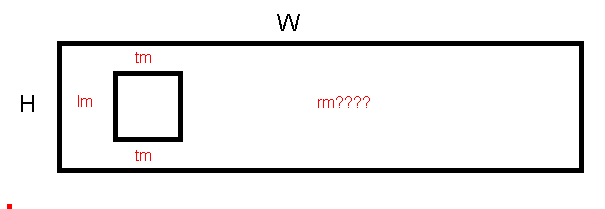# Calculate UIButton InsetsI have a UIButton… it’s frame is W x H in size
I need to insert an Image… with calculated Insets…
The Left (lm) , Top and Bottom ™ are constants
but I need to and cannot figure what the algorithm should be for RIGHT inset (rm)
so that the image comes out square

``````imageEdgeInsets = UIEdgeInsets(top:tm,left:lm,bottom:tm,right:rm)
``````

I need to recalculate TM or LM changes

I could “by guess and by golly” it… but it might need to change programmitcially so that isn’t an option

Height of image = H - 2*tm = Width of Image

→ rm = W - lm - Height of Image

Which is what I’d have thought…

Which should be

``````rm = W-H-(2*tm)
``````

but its not

and actually I would have thought

``````rm = w-(h-(2*tm))-lm
``````

Technically the size of the Image should be

``````h-(2*tm)
``````

Ok… looks like THIS is the equation

``````rm = W-(h-(2*tm))-lm
``````

Because that is NOT the same thing! That should be

``````rm  =  W - ( H - 2*tm ) - lm = W - H + 2*tm - lm
``````

Which is the same thing I wrote …

Its not the same thing you wrote…

you wrote

``````rm = W - lm - h
``````

which isn’t the same as

``````rm = W-(h-(2*tm))-lm
``````

but thanksNo, I did not.

I didn’t write to subtract the height of the canvas H but the Height of Image which I determined in the first line to be

And I like to do it in two lines because it avoids the second error you made.

Because there are TWO mistakes in your original calculations:

(1) you forgot to also subtract lm

(2) you forgot to change the minus to plus when you resolved the brackets, eg

- (H - 2tm) = - H + 2tm